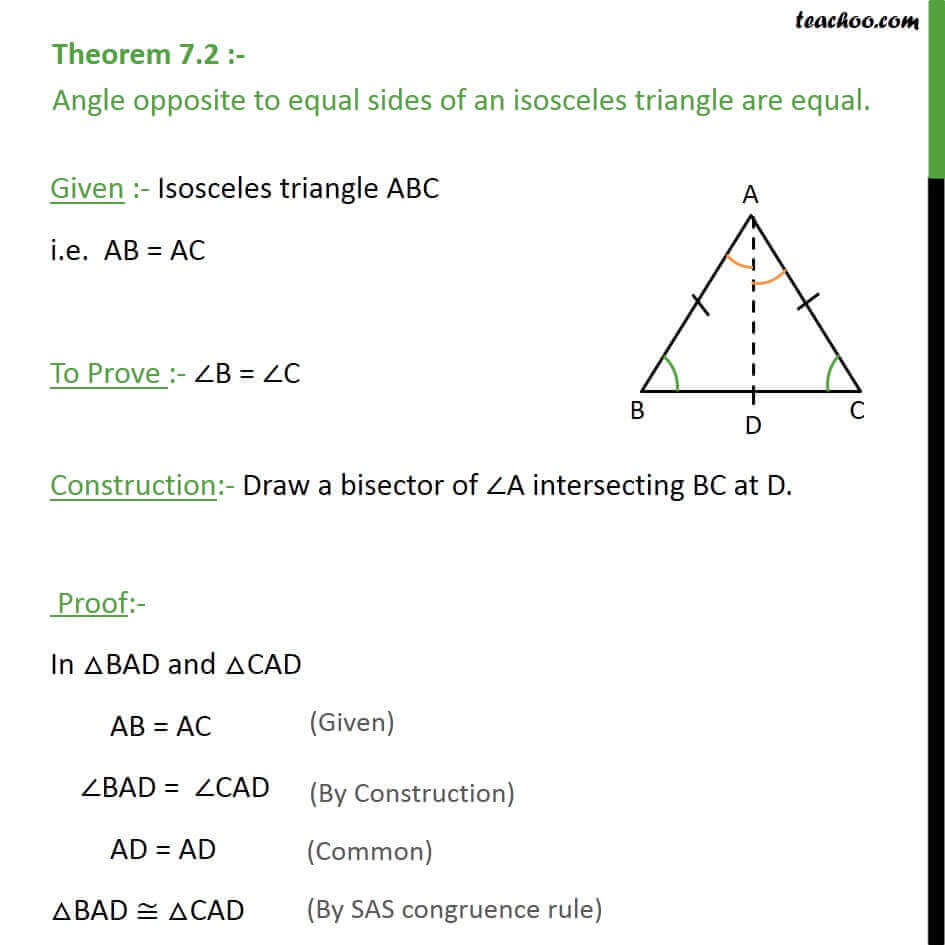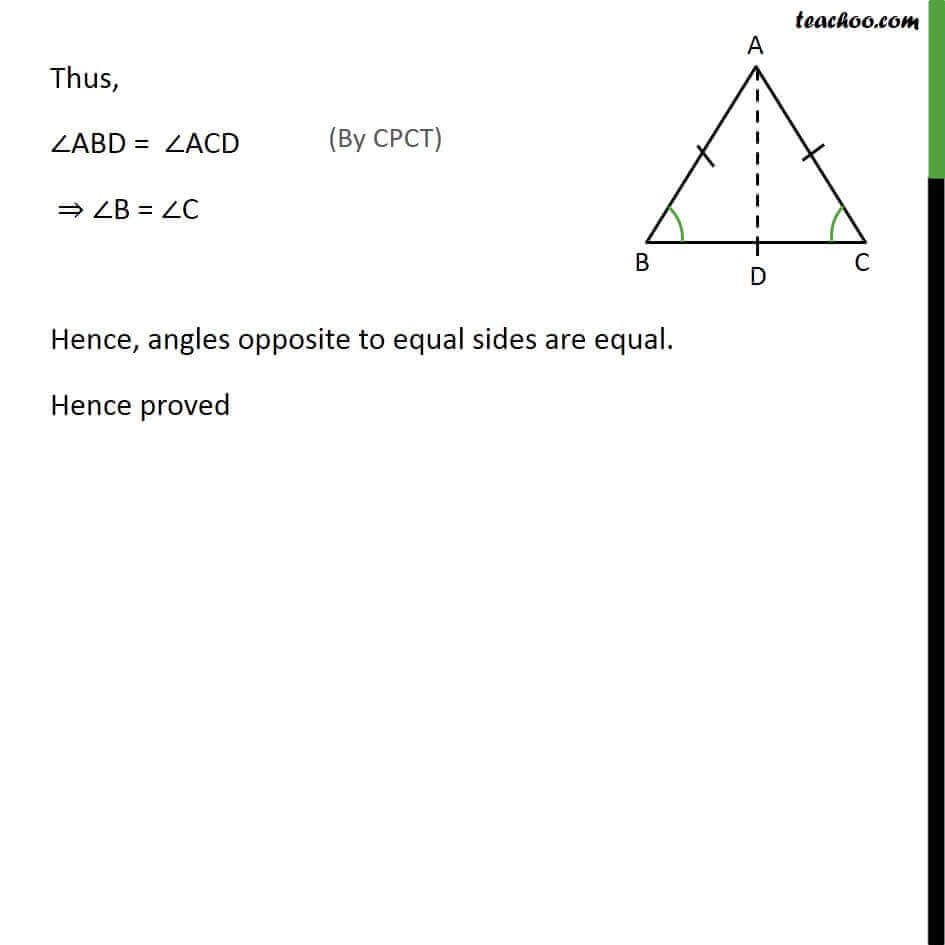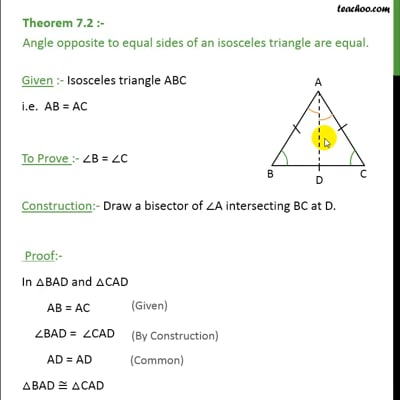Opposite Angles of equal sides

Chapter 7 Class 9 Triangles
Concept wiseThis video is only available for Teachoo black users

Introducing your new favourite teacher - Teachoo Black, at only ₹83 per month

### Transcript

Theorem 7.2 :- Angle opposite to equal sides of an isosceles triangle are equal. Given :- Isosceles triangle ABC i.e. AB = AC To Prove :- ∠B = ∠C Construction:- Draw a bisector of ∠A intersecting BC at D. Proof:- In △BAD and △CAD AB = AC ∠BAD = ∠CAD AD = AD △BAD ≅ △CAD Thus, ∠ABD = ∠ACD ⇒ ∠B = ∠C Hence, angles opposite to equal sides are equal. Hence proved